Courses

# Ratio And Proportion Quant Notes | EduRev

## Quantitative Techniques for CLAT

Created by: Gyanm Institute

## Quant : Ratio And Proportion Quant Notes | EduRev

The document Ratio And Proportion Quant Notes | EduRev is a part of the Quant Course Quantitative Techniques for CLAT.
All you need of Quant at this link: Quant

Chapter - 13

RATIO AND PROPORTION

1) Ratio: The ratio of two quantities a and b in the same unit is the fraction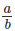and we write it as a: b. In the ratio a: b we call a as the first term or antecedent and b, the second term or consequent.

2) Proportion: The equality of two ratios is called proportion. If a: b = c : d, we write a : b :: c : d and we say that a, b, c, d are in proportion. Here a and d are called extremes while b and c are called means.

Product of means = Product of extremes.

Thus a:b :: c:d implies b x c = a x d

3) Continued proportion: Three numbers a, b and c are said to be in continued proportion if a, b, b, c are in proportion. For example 5, 15 and 45 are in continued proportion because 5:15 :: 15:45

4) Mean proportional and third proportional: If a, b, c are in continued proportion, then b is called the mean proportional between a and c and c is called the third proportional of a and b. Mean proportional between two  numbers is the square root of their product .

Hear mean proportional b = √ac

Some useful results on proportion

If a, b, c and d are four quantities. then

(i) a:b :: c:d means b:a :: d:c  (invertendo)

(ii) a:b :: c:d means a:c :: b:d (Alternando)

(iii) a:b :: c:d means (a+b) : b :: (c+d) : d (Componendo)

(iv) a:b :: c:d means (a - b) : b :: (c - d) :d (dividendo)

(v) If a:b :: c:d then (a + b) : (a – b) :: (c + d) : (c – d) (componendo and dividendo)

(vi) If a:b :: c:d then a: (a – b) :: c : (c – d) (convertendo)

Some useful result for proportional division

The process of dividing a given quantity into parts which are proportional to a given ratio is called proportional division and the resultant quantities obtained are called proportional parts. For example: If 240 is to be divided in the ratio 3:4:5 60, 80 and 100 will be the proportional part since the ratio of these quantities is 3:4:5 and their sum is 240.

Method of finding proportional part

If a quantity P is to be divided in the ratio of a:b:c and the resultant proportional parts are x, y and z then

x = ka, y = kb and z = kc

Now      P = x + y +z

or ka + kb + kc = P

or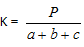Proportional part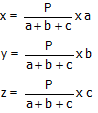So to calculate the proportional parts

a) Given quantity is divided by the sum of ratios.

b) The quotient so obtained is multiplied by the respective numbers of the given ratio.

c) The resultants will be the required proportional parts

For example: Divide 2700 in the ratio 2:3:4

Sum of the ratio = 2 + 3 + 4 = 9

First part =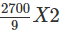= 300 x 2 = 600

Second part =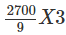= 300 x 3 = 900

Third part =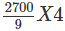= 300 x 4 = 1200

Solved Examples: If 38% of A = 52% of B then A : B = ?

Sol: It means A x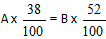or 38 A = 52 B or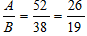. So A : B = 26:19

2) Of the two numbers 40% of first = 60% of second. What is the proportion of first number to second

Sol: The ratio of two numbers

or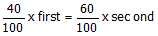or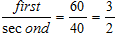3) The salaries of A, B and C are in the ratio 3:5:7. If their salaries are increased by 50%, 60% and 50% respectively, the ratio between new salaries is

Solution: After increase of 50% Salary of A is 3 X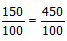After increase of 60% of salary of B is =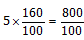After increase of 50% salary of C is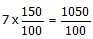Ratio between the new salaries of A, B and C is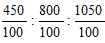or 45:80:105      or 9:16:21

4) In a School 10% of the total no. of boys is equal to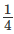of the total number of girls. The ratio between number of boys and number of girls.

Solution: Let B be total no. of boys and G is the total number of girls.

As per the given condition in the question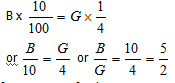So No of Boys to No of girls = 5: 2

5) The speed of three Cars is in the ratio of 3:4:5. The ratio between the time taken by them to travel a fixed distance is

Solution: Let fixed distance be d

Time taken by first car is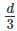Time taken by second car is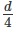Time taken by 3rd car is =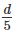So ratio of time between three cars is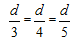or ratio is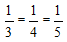or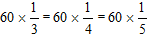20 : 15 : 12

6) Total number of boys in a school are 16% more than the total number of girls in the school. What is the ratio between total no. of boys to total girls

Solution: Let B be total number of boys and G the total number of girls.

As per the given condition in the question

B = G + 16% of G

or B = G +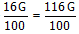or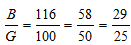So Ratio of B to G is 29:25

7) A sum of money is to be divided amongst P, Q and R in the ratio 5:6:7. If Q got Rs 2100 more than P, how much amount did Q receive

Solution: The ratio of P and Q is 5:6

So if Q gets Rs 1 more than P, Q gets Rs 6

If Q gets Rs 2100 more than P, then Q gets

2100 x 6 = Rs12600

8) Total number of students in a school is 1224. If the number of girls in the school is 600, then what is the ratio between the total number of boys to total number of girls

Solution: Total students in the school = 1224

Total no. of girls in the school = 600

So Total no. of boys in the school = 1224 – 600=624

Ratio of boys to girls

624 : 600

or 156 : 150

or  78 : 75

or  26 : 25

9) A sum of money is to be divided amongst A, B, C and D in the ratio 3:7:9:13. If the share of B is Rs 4872 then what is the total amount of money of A and C

Solution: Ratio amongst A : B: C: D is 3 : 7 : 9 : 13

From this ratio If B gets Rs 7, A and C get Rs 12

If B gets Re 1, A and C will get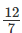If B gets R 4872, A & C will get =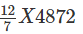= 12 x 696

= 8352

10) There are 270 students in a school. The ratio of number of boys to number of girls studying in the school is 25:29. If 15 boys and 15 girls take admission in the school., what will be the new ratio of boys to girls.

Boys   Girls

Solution: Let us divide 270 in the ratio 25 :    29

Boys =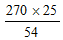= 125  Girls = 29 X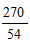= 145

15 boys and 15 girls take admission

Now no. of boys = 140

No of girls = 160

Ratio 140 : 160 or 7 : 8

11) A mixture contains alcohol and water in the ratio 3:2. On adding 5 litre of water in it, the quantities of alcohol and water become equal. What is the quantity of alcohol in the mixture.

Solution: Ratio of alcohol to water is 3 : 2. If 3 litre is alcohol then 2 litre is water

If we add one litre of water the quantity of alcohol and water become equal

So if on adding 1 litre of water quantities become equal then quantity of alcohol is 3 litre

If on adding 5 litre they become equal then alcohol is 3 x 5 = 15 litre.

12) When a particular number is subtracted from each of 7, 9, 11 and 15, the resulting numbers are in proportion. What is the number to be subtracted

Solution: Let the number to be subtracted is x

Then (7 – x) (15 – x) = (9 – x) (11 – x)

or 105 – 7x – 15x + x2 = 99 – 9x – 11x + x2

or -22x + 105 = -20x + 99

or  6 = 2x  or x = 3

13) Two metals contain zinc and copper in the ratio 2:1 and 4:1 respectively. In what ratio these two must be mixed to get a new metal containing zinc and copper in the ratio 3 : 1

Solution: By the method of allegation and mixture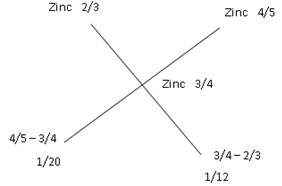By the method of allegation and mixture

The reqd. ratio is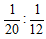Or 3:5 [multiply by 60]

14) Mean proportional between 104 and 234 is

Solution:  Mean proportional is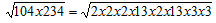= 2x2x3x13= 156

15) Fourth proportional to 0.2, 0.12 and 0.3 is

Solution: Let fourth proportional be x

So 0.2 x = 0.12 x 0.3

or x =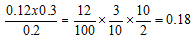16) Third proportional to 0.36 and 0.48 is

Solution: Third proportional is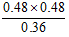= 0.64

17) Four milk men rented a pasture. A grazed 18 cows for 4 months B 25 cows for 2 months, C 28 cows for 5 months and D 21 cows for 3 months. If A’s share of rent is Rs 360, total rent of the field is

Solution:

A – 18 X 4 = 72 cow month

B – 25 x 2 = 50 cow month

C – 28 x 5 = 140 cow month

D – 21 x 3 = 63 cow month

A’s cow months and total cow months are in the ratio 72 : 325, rent will also be in this ratio.

If A’s rent is 360 then total rent =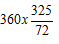= 1625

18) There are three partners A, B and c in a firm. A’s capital is equal to thrice of B’s and B’s capital is 4 times C’s capital. Find the ratio of the capital of A, B and C

Solution: Given is A = 3B

and B = 4c

so A = 3B = 3 x 4c

So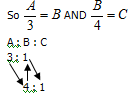So Ratio of A : B : C is 12 : 4 : 1

19) A and B enter into partnership with capitals as 5 : 6. At the end of 8 months A withdraws. If they receive profit in the ratio of 5 : 9, find how long B’s capital was used.

Solution: Since profit is in the ratio 5 : 9, So capital will also be in the same ratio.

Money invested is in the ratio 5 : 6 and A’s capital was used for 8 months. So 8 x 5 is 40

Since ratio of profit is 5 : 9 So capital will be in the ratio 5 : 9 if B’s capital is 72

So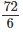months

So B’s capital was used for 12 months

20) In a mixture of 75 litres, the ratio of milk to water is 2:1. How much water should be added in the mixture so that the ratio of milk to water is 1 : 2

Solution: Ratio of milk to water is 2 : 1

In 75 litres milk is 50 and water is 25 i.e. 50:25

We want to make the ratio as 1 : 2 i.e 50 : 100.

Since the milk in two ratios is 50 each and we want to convert 25 water to 100 water. So 75 litres of water be add.

## Quantitative Techniques for CLAT

63 videos|22 docs|93 tests

,

,

,

,

,

,

,

,

,

,

,

,

,

,

,

,

,

,

,

,

,

;